## Table of ContentOpen Access

ARTICLE

# Assessing Criteria Weights by the Symmetry Point of Criterion (Novel SPC Method)–Application in the Efficiency Evaluation of the Mineral Deposit Multi-Criteria Partitioning Algorithm

Zoran Gligorić, Miloš Gligorić*, Igor Miljanović, Suzana Lutovac, Aleksandar Milutinović

Faculty of Mining and Geology, University of Belgrade, Belgrade, 11 000, Serbia

* Corresponding Author: Miloš Gligorić. Email:(This article belongs to this Special Issue: Advanced Computational Models for Decision-Making of Complex Systems in Engineering)

Computer Modeling in Engineering & Sciences 2023, 136(1), 955-979. https://doi.org/10.32604/cmes.2023.025021

## Abstract

Information about the relative importance of each criterion or the weights of criteria can have a significant influence on the ultimate rank of alternatives. Accordingly, assessing the weights of criteria is a very important task in solving multi-criteria decision-making problems. Three methods are commonly used for assessing the weights of criteria: objective, subjective, and integrated methods. In this study, an objective approach is proposed to assess the weights of criteria, called SPC method (Symmetry Point of Criterion). This point enriches the criterion so that it is balanced and easy to implement in the process of the evaluation of its influence on decision-making. The SPC methodology is systematically presented and supported by detailed calculations related to an artificial example. To validate the developed method, we used our numerical example and calculated the weights of criteria by CRITIC, Entropy, Standard Deviation and MEREC methods. Comparative analysis between these methods and the SPC method reveals that the developed method is a very reliable objective way to determine the weights of criteria. Additionally, in this study, we proposed the application of SPC method to evaluate the efficiency of the multi-criteria partitioning algorithm. The main idea of the evaluation is based on the following fact: the greater the uniformity of the weights of criteria, the higher the efficiency of the partitioning algorithm. The research demonstrates that the SPC method can be applied to solving different multi-criteria problems.

## Graphical Abstract## Keywords

Nomenclature

 MCDM Multi-criteria decision-making SPC Symmetry point of criterion DM Decision matrix ADM Attribute decision matrix TMC Technological mining cut MCAV Mining cut attribute vector BAV Block attribute vector EOA Efficiency of the algorithm

1  Introduction

Multi-criteria decision-making (MCDM) refers to ranking the given set of alternatives with respect to the given set of criteria. Assessing the weights of criteria is a very important phase in most MCDM models. The assessment of the weights of criteria significantly influences the final rank of alternatives. Various methods have been developed to determine the weights of the criteria. The process of the criteria weight determination plays a key role in every multi-criteria decision-making (MCDM) problem. The high impact of the criteria weights is directly reflected on the final ranking i.e., optimal final decision. Generally, methods for criteria weight determination are divided into three groups, such as subjective weighting methods, objective weighting methods and combined weighting methods.

Many authors have used objective weighting methods to obtain the final ranking of alternatives. Krishnan et al.  developed a modified procedure of the CRiteria Importance Through Intercriteria Correlation (CRITIC) method, called the Distance Correlation-based CRITIC (D-CRITIC) method. They applied five smartphone models to evaluate the criteria weights by D-CRITIC. The obtained results were compared with four other objective weighting methods and analyzed by three different tests to validate the performance of the D-CRITIC method. Žižović et al.  presented a new way for modification of the CRiteria Importance Through Intercriteria Correlation (CRITIC) method, called CRITIC-M. The modification was composed of two approaches. The first referred to the normalization technique of the initial data and the second to the expression for determining the final values of the criteria weights. Cavallaro et al.  proposed a fuzzy multi-criteria model for combined heat and power (CHP) systems selection. The developed model was based on a fuzzy Shannon’s entropy method to compute the objective weights of the criteria while a fuzzy Technique for Order of Preference by Similarity to Ideal Solution (TOPSIS) method was applied for the final ranking of the alternatives (CHP technologies). Wang et al.  presented a sustainable battery supplier selection for battery swapping stations based on MULTIMOORA method with uncertainty. The uncertainty was expressed as triangular fuzzy numbers describing the behavior of the input data. The weights of criteria were evaluated by the entropy method. They also performed the verification of the proposed methodology through the sensitivity analysis and comparative analysis with other MCDM methods in a case study in Beijing, China. Chodha et al.  utilized the TOPSIS technique to select the best industrial robot for arc welding. The eight possible alternatives (robots) with respect to the five criteria were taken for the final decision. The entropy weighting method was used to denote the objective criteria weights. Vavrek et al.  applied three objective weighting methods (Coefficient of variation–CV, Standard deviation–SD and Mean weight–MW) integrated with the TOPSIS method for assessing the efficiency of cultural services in the Czech Republic. They represented 26 theatres (alternatives) related to 11 indicators (technical and financial) to evaluate the efficiency of the theatres. Mukhametzyanov  presented three objective weighting methods (Entropy, CRITIC and SD) for criteria weight determination and performed a comparative analysis of them. Vujičić et al.  suggested a multi–criteria decision–making method for air conditioner selection. They applied two MCDM methods, MOORA and SAW, to select the best alternative, while the criteria weights were determined by two objective weighting methods, Entropy and CRITIC. The numerical example was expressed through the case study to illustrate a comparison analysis of the combined MCDM methods and objective weighting approaches. Yalcin et al.  examined several different measures, such as accounting-based performance (ABP), value-based performance (VBP) and overall performance (OP), to evaluate the performance of initial public offering (IPO) firms in the pre-IPO and post-IPO periods. The VIKOR method was utilized for ranking the IPO firms, while objective weighting methods (CRITIC and MW) were applied to determine the weights of criteria. They displayed the results by case study to validate the performance of 16 Turkish IPO firms. Şahin  investigated the material selection problem for a flywheel. The grey relational analysis (GRA), TOPSIS and Organization Rangement Et Synthese De Donnes Relationnelles (ORESTE) method were employed for the final ranking of the materials. The objective weighting methods, SD and CRITIC method, were implemented to establish the significance of criteria. The six approaches based on MCDM methods combined with objective weighting methods were compared and integrated with the Copeland method to choose the best alternative. Wei et al.  proposed the extended GRA method for solving the probabilistic uncertain linguistic multiple attribute group decision-making (MAGDM) with unknown attributes (criteria) information. The CRITIC method was used to calculate the objective criteria weights. They were shown a numerical example for site selection of electric vehicle charging stations (EVCS) to verify the developed algorithm. Mešić et al.  created a new hybrid model based on a combination of the CRITIC and MARCOS methods for the estimation of the logistics performance index in Western Balkan countries. The weights of six criteria were analysed and determined by the CRITIC method and then the five alternatives (Western Balkan countries) were ranked by the MARCOS method. Keshavarz-Ghorabaee et al.  developed a novel method for criteria weight determination, namely MEREC (MEthod based on the Removal Effects of Criteria). They showed and described a detailed computational analysis of the MEREC method step by step. They also presented a comparative analysis with other objective weighting methods for the validation of the introduced method’s results through the example. Hadi et al.  proposed a combination of the MEREC method and modified TOPSIS method to select the best hospital location for COVID-19 infected patients demonstrated in a real-world case study in Baghdad, Iraq. They showed two main phases of the developed model in which the first phase contains the Internet of Things (IoT) platform represented by geolocation alternatives sites. In contrast, the second phase implements the MCDM techniques represented by a web application system. There are also objective weighting methods that are based on the entropy method, such as Criteria Impact Loss (CILOS) and Integrated Determination of Criteria Weight (IDOCRIW) .

The subjective weighting methods are widely used to evaluate the importance of criteria in MCDM processes. There are many subjective methods, but only a few will be mentioned in the following literature. Gorcun et al.  developed a novel integrated fuzzy model based on fuzzy SWARA and fuzzy CODAS methods for assessing an acceptable road tanker vehicle. They established a set of thirteen criteria and four possible alternatives. Fuzzy SWARA was used to quantify the weights of criteria, while fuzzy CODAS was applied to rank the set of alternatives. Cakar et al.  proposed a model for supplier selection in a dairy company based on the fuzzy TOPSIS method. They adopted ten criteria and six supplier cities as a set of alternatives and created an MCDM problem. The weights of criteria were determined by the subjective assessment of the decision-maker using a linguistic approach. The supplier cities (alternatives) were selected by a fuzzy TOPSIS method. Pamučar et al.  developed a new subjective weighting method for determining the criteria weights named the Full Consistency Method (FUCOM). Stanujkic et al.  proposed a new approach for criteria weights evaluation called PIvot Pairwise RElative Criteria Importance Assessment (PIPRECIA). Keršulienė et al.  presented a new subjective approach for criteria weight determination, which is known as step-wise weight assessment ratio analysis method (SWARA). Krylovas et al.  introduced a novel KEmeny Median Indicator Ranks Accordance (KEMIRA) method for establishing the attribute preferences and to calculate the criteria weights. Besides, the previous mentioned subjective weighting methods, there are some that should be noted, such as AHP , Ranking method , SMART , WEBIRA , OPA  and many others.

Besides, the objective and subjective weighting methods, combined (integrated) weighting methods take an important place in the process of assessing criteria weights. Many authors deal with these approaches for determining the criteria weights. Odu  presented an overview of the basic weighting methods for estimating criteria weights, including a detailed description of the subjective, objective and combined (integrated) weighting methods. Jahan et al.  proposed a framework, including all types of weighting methods for material selection problems. They applied objective and subjective weighting methods to develop a novel combined weighting method for criteria weight evaluation. Chen  combined AHP and Entropy into one combined weighting method integrated with the TOPSIS method to create an MCDM model for the building material supplier selection. Ali et al.  used an integrated weighting method based on a best-worst method (BWM) as subjective and IDOCRIW as objective weighting methods in the decision-making process for power generation technologies selection. Zavadskas et al.  combined objective (entropy, CILOS and IDOCRIW) and subjective (experts’ attitudes) weighting methods to obtain an overall (integrated) criteria weight.

Bearing in mind these traditional and well-known MCDM methods that are widely applied in different areas, there are many approaches that provide support to decision-makers by integrating all types of weighting methods with other methods under uncertain environments, such as MARCOS , MAIRCA , RAFSI , MABAC , MICMAC , TODIM , picture fuzzy set [40,41], rough set theory , neutrosophic set [42,43], intuitionistic set , soft set theory  and many other extensions of these methods.

As can be seen from the comprehensive literature review, no authors deal with weighting methods for the purpose of evaluating the efficiency of some partitioning algorithm. Specifically, there is no paper to estimate the efficiency of the mineral deposit partitioning algorithm with the help of weight coefficients. These methods are mostly used for the classical determination of the weights of criteria in different real-life problems within the MCDM framework. Guided by that fact, we tried to develop a completely new approach. The paper presents a novel method for objectively defining the weights of criteria. The objective method of determining the weights of criteria helps decision-makers to reduce the subjectivity in that complex task and to increase the accuracy of the final results. It should be noted that this novel method represents a new approach to estimating and analyzing the efficiency of the multi-dimensional partitioning algorithm. For that purpose, the tendency is to balance all dimensions (criteria) to obtain more efficient results from the partitioning algorithm.

The main aim of this study is to present the methodology of assessing criteria weights based on the characteristic of the criterion that is expressed by its symmetry. The developed method belongs to the class of objective methods. Symmetry Point of Criterion (SPC) is used to calculate the weights of criteria. The symmetry point is located in the middle of the interval [a, b], where a and b present the lower and upper value of the criterion, respectively. Measuring the absolute distance from every criterion value to the symmetry point of the criterion, we create the absolute distance vector. The set of these vectors is presented in the form of a matrix of absolute distances. The modulus for a primal matrix element is defined as the ratio of the averaged absolute distance of the criterion to a value of the criterion element. In this way we form the matrix of moduli of symmetry. Averaging every column of this matrix, we obtain row vector, where every element represents the modulus of symmetry of the given criterion. Finally, the weight of the criterion is calculated by dividing every element of the row vector by the sum of the elements.

The application of the developed method is demonstrated to evaluate the efficiency of the mineral deposit partitioning algorithm. This algorithm presents the base for mine production planning. The main objective of the mineral deposit partitioning algorithm is to divide a deposit into a predefined number of parts, and each part should meet the requirements made up by production planners. Usually, these requirements are presented by the elements of the technological vector. The efficiency of the algorithm can be viewed as a multi-criteria decision-making problem. In that context, part of the mineral deposit can be treated as an alternative, while technological requirements are treated as criteria. If we take into consideration that each part should have equal values to the technological requirements (technological criteria), it implies the uniformity of the weights of criteria. Optimal partitioning means the equal values of the weights of criteria. In that case, the entropy of the criteria weights vector has the maximum value. Making the comparison between the entropy of the optimal partitioning and real-life partitioning, we can calculate the efficiency of the algorithm.

In this study, after presenting the theory of the proposed method, we illustrate the method through an artificial multi-criteria decision-making problem. A comparative analysis with three objective weights methods (CRITIC, Entropy, Standard Deviation and MEREC) has also been undertaken in Section 3, and the results show that the SPC method is very efficient in assessing the weights of criteria objectively. The applicability of the SPC method in the solving real-life problems is demonstrated in Section 4, where the efficiency of the mineral deposit multi-criteria partitioning algorithm is calculated by using the entropy of the weights of criteria. Section 5 presents the conclusion.

2  The SPC Method

In this section, a new method based on the Symmetry Point of Criterion (SPC) is proposed for assessing the weights of criteria in any multi-criteria decision-making problem. The SPC method uses the symmetry point of criterion, i.e., the modulus of symmetry of the criterion to measure its influence on the weights of criteria. A higher value of the modulus indicates a greater weight of the criterion. The following steps are used to estimate the weights of criteria by objective SPC method.

Step 1: Create the decision matrix

Suppose that there are two finite sets, A and C. Set A considers available alternatives, while set C considers criteria that will be used to assign adequate values to the alternatives. Let m and n denote a total number of alternatives and criteria, respectively. Accordingly, the decision has the following form:

DM=|xij|m×n=|A/CC1C2CnA1x11x12x1nA2x21x22x2nAmxm1xm2xmn|(1)

where:

xij–an assessment of alternative Ai with respect to a set of criteria

m–total number of alternatives

n–total number of criteria

Step 2: Calculate the Symmetry Point of Criterion (SPCj)

In computing the symmetry point of the criterion only extreme values should be considered. Let Xi1={x11,x21,,xi1}T;i[1,m] be a column vector of C1 criterion values, with respect to a set of alternatives. If the lower and upper values of the interval [a, b] are defined as a=min{x11,x21,,xi1}T and b=max{x11,x21,,xi1}T, respectively, then the point c, which is located in the middle of the interval, represents the symmetry point of criterion C1. The common equation for calculation the symmetry point is as follows:

SPCj=min{xij}+max{xij}2;i=1,2,,m;j[1,n](2)

Step 3: Create the matrix of absolute distances

Recall vector Xi1 and create new extended vector by inserting an SPC1 point at the specified location. For example, Xi1={x11,x21,,SPC1,,xi11,xi1}T;i[1,m] represents an extended vector. Obviously, some values of the criterion are located on the left side of SPC1, and some on the right side. Further, the absolute distance from every criterion value to SPC1 should be calculated: {|x11SPC1|,|x21SPC1|,,|xi11SPC1|,|xi1SPC1|}T;i[1,m]. If we take into consideration a primal decision matrix, then the matrix of absolute distances can be represented in the following way:

D=||dij||m×n=||x11SPC1||x12SPC2||x1nSPCn||x21SPC1||x22SPC2||x2nSPCn||xm1SPC1||xm2SPC2||xmnSPCn||(3)

Step 4: Create the matrix of the moduli of symmetry

Let Di1={d11,d21,,di1}T;i[1,m] be the column vector of the absolute distances concerning criterion C1. The modulus for a primal matrix element x11 is defined as the ratio of the averaged absolute distance of criterion C1 to a value of element x11: i=1mdi1m/x11. Therefore, the matrix of the moduli of symmetry is of the following form:

R=|rij|m×n=|i=1mdi1mx11i=1mdi2mx12i=1mdinmx1ni=1mdi1mx21i=1mdi2mx22i=1mdinmx2ni=1mdi1mxm1i=1mdi2mxm2i=1mdinmxmn|(4)

Step 5: Calculate the modulus of symmetry of criterion

Averaging every column of the previous matrix R, we obtain row vector Q, where every element q represents the modulus of symmetry of the jth criterion. Vector Q is defined as:

Q=|q1j|1×n=|i=1mri1mi=1mri2mi=1mrinm|;j[1,n](5)

Step 6: The weights of criteria

Finally, in this step, each objective criterion weight is computed using the vector of moduli of symmetry. The following equations are used for assessing the weights of criteria:

W=|w1j|1×n=|q1j=1nqjq2j=1nqjqjj=1nqj|(6)

Fig. 1 shows the flowchart of the SPC method.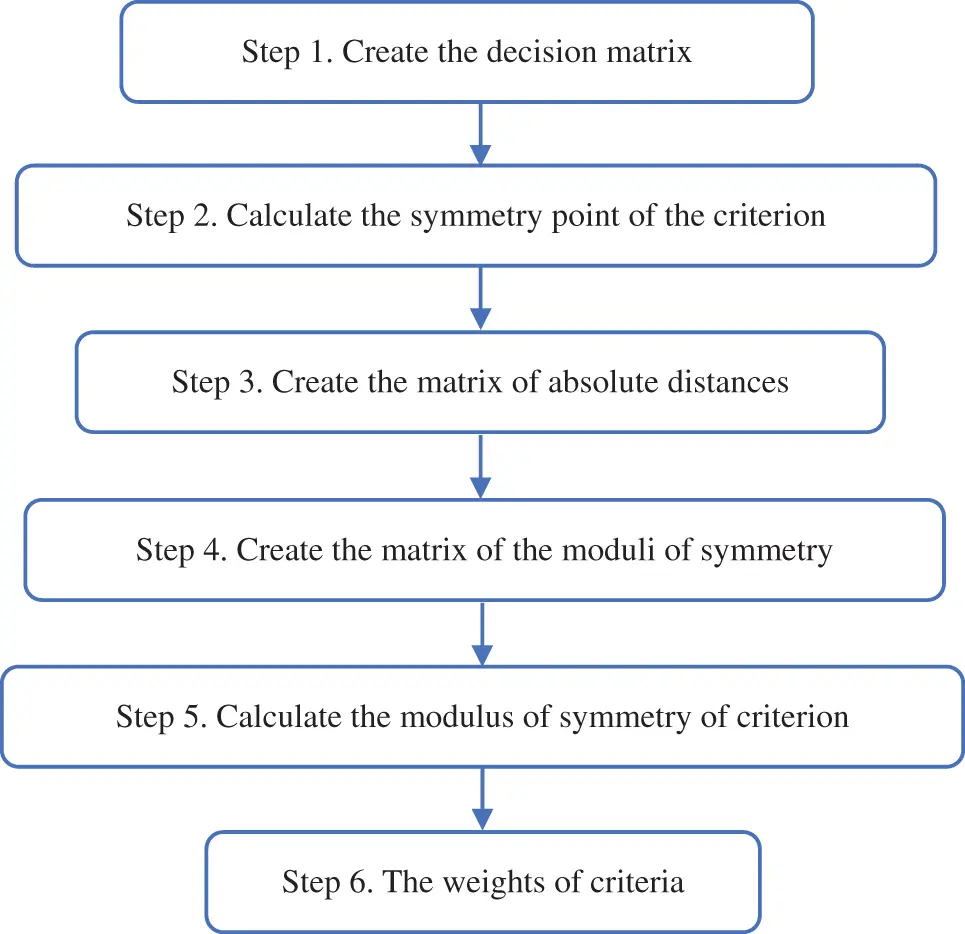Figure 1: The flowchart of the SPC method

3  The Efficiency of the Mineral Deposit Multi-Criteria Partitioning Algorithm by SPC Method

Underground mining experts are faced with an extremely hard task concerning production planning with respect to the technological requirements. Geostatistical methods use data obtained by exploration drilling to create a block model of a mineral deposit. A mineable block is a three-dimensional object which can be mined in an economically viable way. Each block is characterized by the following attributes: specified dimensions (for example: 10 m × 10 m × 10 m), ore tonnage (for example: 2200 t), ore grades (for example: 1.75% Lead; 3.79% Zinc), and specified x, y, and z coordinates.

The authors borrowed a mineral deposit partitioning algorithm from Gligorić et al.  to evaluate its efficiency through the developed SPC method. A hypothetical example borrowed from Gligorić et al.  is analysed in the case study. It represents the multi-criteria partitioning algorithm for a mineral deposit. Our idea and motivation of the new proposed method are directed towards the validation of the developed partitioning algorithm. Since the algorithm divided the mineral deposit into several areas that must meet certain technological requirements, we tried to verify these areas by a new approach based on the objective weighting method. The efficiency of our partitioning algorithm is manifested as a uniform distribution of weight coefficients. The new proposed SPC method has proved that the mineral deposit partitioning algorithm succeeded in dividing the mineral deposit to a very high-level, considering the target values of the multi-dimensional TMCs.

A mineral deposit can be presented by a finite set of mineable blocks B={bh}h[1,H], where H is the total number of mineable blocks. Characteristics of the blocks are expressed by an attribute vector BAVh={ajh}j[1,A],h[1,H], where A is the total number of attributes.

On the other hand, a technological mining cut (TMC) is defined as a subset of B; TMCi={bli}l<H;i[1,N], where l denotes a total number of blocks associated to the ith TMC, and N denotes a total number of TMCs. Obviously, TMC is a union of mineable blocks (the homogeneous part of a mineral deposit) characterized with respect to a given set of technological requirements. The total number of technological mining cuts equals the number of mining periods (years of mining). Technological requirements are defined by mine planners and can be presented by the vector C={Cj}j[1,K], where K denotes the total number of requirements. Each TMC can be described by a mining cut attribute MCAVi={aic}c=1,2,..,K;i[1,N], where K is the total number of attributes, and equals the number of requirements.

The creation of TMCs can be formulated as a multi-objective optimization problem. To solve this problem, Gligorić et al.  developed a multi-criteria clustering algorithm based on the maximization of the similarity between vector MCAVi={aic}c=1,2,..,K;i[1,N], and Cteh={cjteh}j[1,K], where Cteh represents the required technological vector. A solution of the formulated problem is given in the following form:

B=i=1NTMCi;TMCαTMCβ=;αβ(7)

Accordingly, we can draw an analogy between the MCDM problem and mineral deposit partitioning. Technological mining cuts can be treated as alternatives and require a technological vector as a vector of criteria. This analogy can be presented by the following decision matrix:

where

zij–a value of the ith TMC with respect to the jth technological criteria.

The main idea of the efficiency evaluation of the mineral deposit partitioning algorithm is based on the following theorem:

Theorem: for a discrete uniform probability density function p on a finite set of the weights of criteria (w1,w2,,wj),wj0,j=1Kwj=1, entropy has the maximum value:

H({Wj}j[1,K]U(w1,w2,,wj))max(9)

In terms of algorithm efficiency, the theorem can be formulated as: the greater the uniformity of the weights of {c1teh,c2teh,,cKteh} criteria, the higher the efficiency of the partitioning algorithm. It means that the weights of criteria should be equal as far as possible.

Proof: The divergence between two discrete distributions p and q is defined as :

D(p||q)E[logp(Wteh)q(Wopt)](10)

where

p(Wteh)–discrete distribution of the weights of the technological criteria realized by the SPC method.

q(Wopt)–discrete distribution of the optimal weights of the technological criteria.

Further, U denotes the discrete uniform distribution on Wopt={w1opt,w2opt,,wjopt},j[1,K], while p denotes an arbitrary discrete distribution on Wteh={w1teh,w2teh,,wjteh},j[1,K]. Entropy, for a probability density function p on a finite set (w1,w2,,wj),wj0,j=1Kwj=1, is defined by the Shannon equation :

H(p(w1,w2,,wj))=j=1Kp(w1,w2,,wj)log(p(w1,w2,,wj))(11)

The non-negativity of relative entropy implies that:

D(p||U)0(12)

Hence,

D(p||U)=j=1Kp(wj)logp(wj)U(wj)=j=1Kp(wj)log(p(wj))j=1Kp(wj)log(U(wj))(13)

D(p||U)=H(p(w1,w2,,wj))j=1Kp(wj)log(U(wj))(14)

Note that U(wj)=1K,j[1,K]. Obviously, U(wj) is a constant, and we can pull it out of the summation and rewrite the previous equation as:

D(p||U)=H(p(w1,w2,,wj))log(U(wj))j=1Kp(wj)(15)

According to the constraint j=1Kp(wj)=1, we can substitute 1 for expression j=1Kp(wj). Since 1 is also a probability distribution, the following equality holds:

1=j=1K(U(wj))(16)

This substitution gives us:

D(p||U)=H(p(w1,w2,,wj))log(U(wj)j=1K(U(wj)))=H(p(w1,w2,,wj))+H(U(w1,w2,,wj))(17)

where H(U(w1,w2,,wj)) defines the entropy of the uniformly distributed optimal weights of the technological criteria.

According to the above discussion, we have shown that:

D(p||U)=H(p(w1,w2,,wj))+H(U(w1,w2,,wj))(18)

Applying the fact that D(p||U)0 we can conclude that:

H(U(w1,w2,,wj))H(p(w1,w2,,wj))(19)

If we take into consideration that the probability distribution on Wteh={w1teh,w2teh,,wjteh},j[1,K] was arbitrary, the proof is completed. Thus, it means the theorem: “the greater the uniformity of the weights of criteria, the higher the efficiency of the partitioning algorithm”, is valid and can be used to compute the efficiency of the algorithm.

The efficiency evaluation of the algorithm is performed through the following steps:

Step 1: Calculate the weights of criteria by the application of the SPC method over the ADM matrix data, and present them through the following vector:

Wteh={wjteh}j[1,K]={w1teh,w2teh,,wKteh}(20)

Step 2: Calculate the optimal values of the weights of criteria as follows:

Wop={wjopt}j[1,K]={w1opt=w2opt==wjopt=1K}j[1,K](21)

Step 3: Compute the entropy of the calculated weights. Shannon  developed an entropy theory based on thermodynamic that can be interpreted as the quantity of information needed to define the physical state of a system. Entropy can be considered as a measure of the degree of information regularity in weights. The amount of information related to the weights of criteria are calculated using the equations below:

Hteh=j=1Kwjtehlog(wjteh)(22)

Hopt=j=1Kwjoptlog(wjopt)(23)

Step 4: Calculate the efficiency of the algorithm (EOA). Discussion about the formulated theorem indicates that entropy of the optimal values of the weights of criteria can be set up as a target value. Based on this, the efficiency of the partitioning algorithm can be calculated as follows:

EOA=HtehHopt×100%(24)

where

Hteh–entropy of the technological weights of criteria obtained by the SPC method.

Hopt–entropy of the optimal weights of criteria (target value).

Transformation of the numerical values of the EOA to the linguistic variables is presented in Table 1.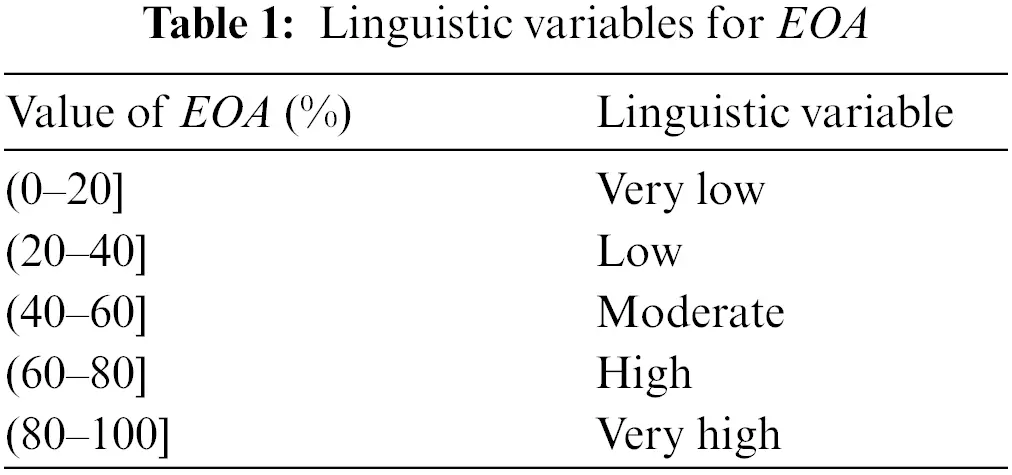The required technological vector Cteh is composed of the following components:

c1teh–tonnage of a TMC.

c2teh–compactness (homogeneous) of a TMC, expressed by Schwartzberg’s index ; it is defined as the ratio of the square perimeter of the shape to the area.

c3,γteh–standard deviation of the ore grade in a TMC with respect to the type of a metal hosted in a deposit (%). If γ2 then deposit is polymetallic.

A brief description of the data needed to execute the partitioning algorithm is presented in Tables 2, 3, and Fig. 2 (for more details see ).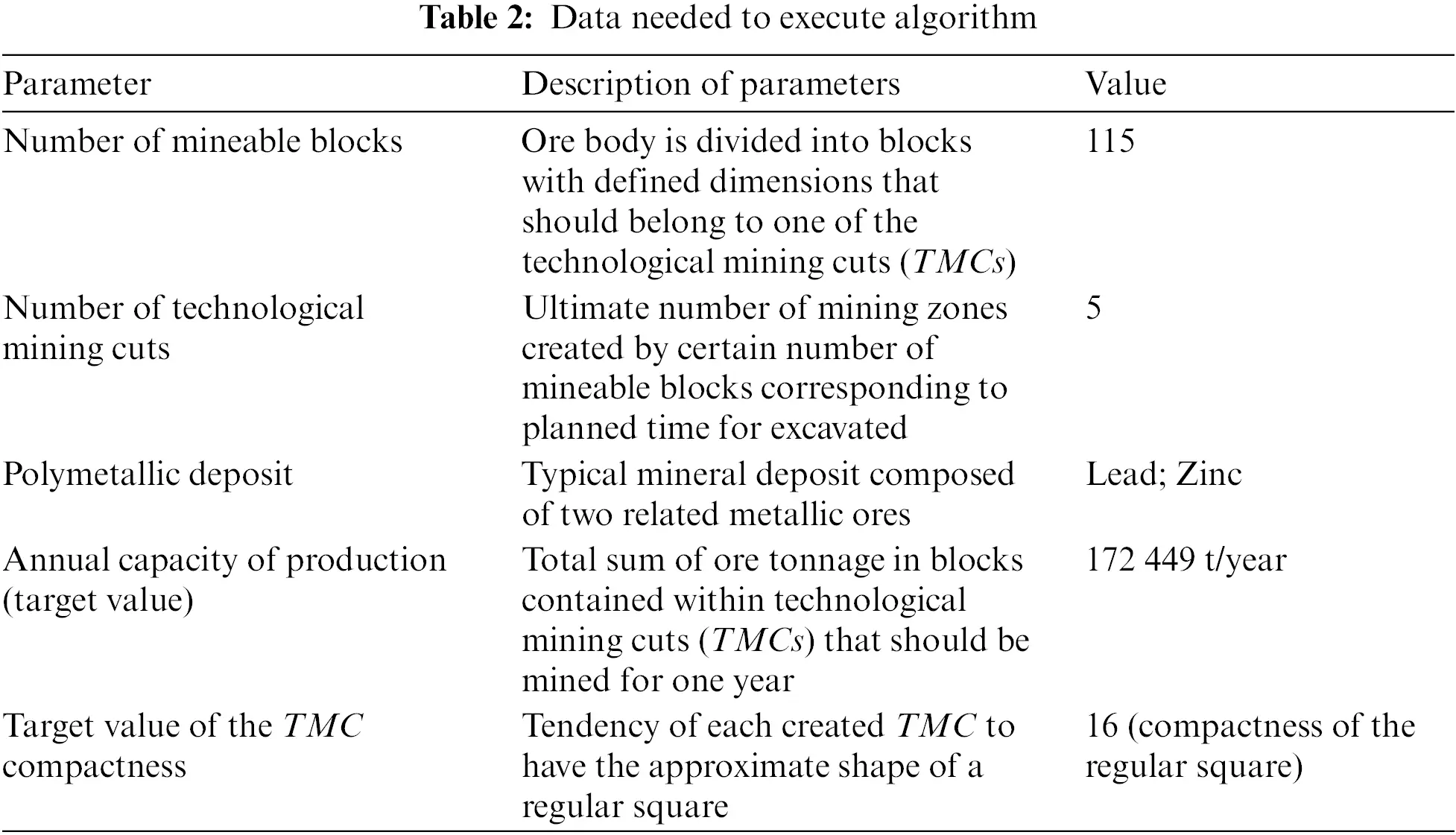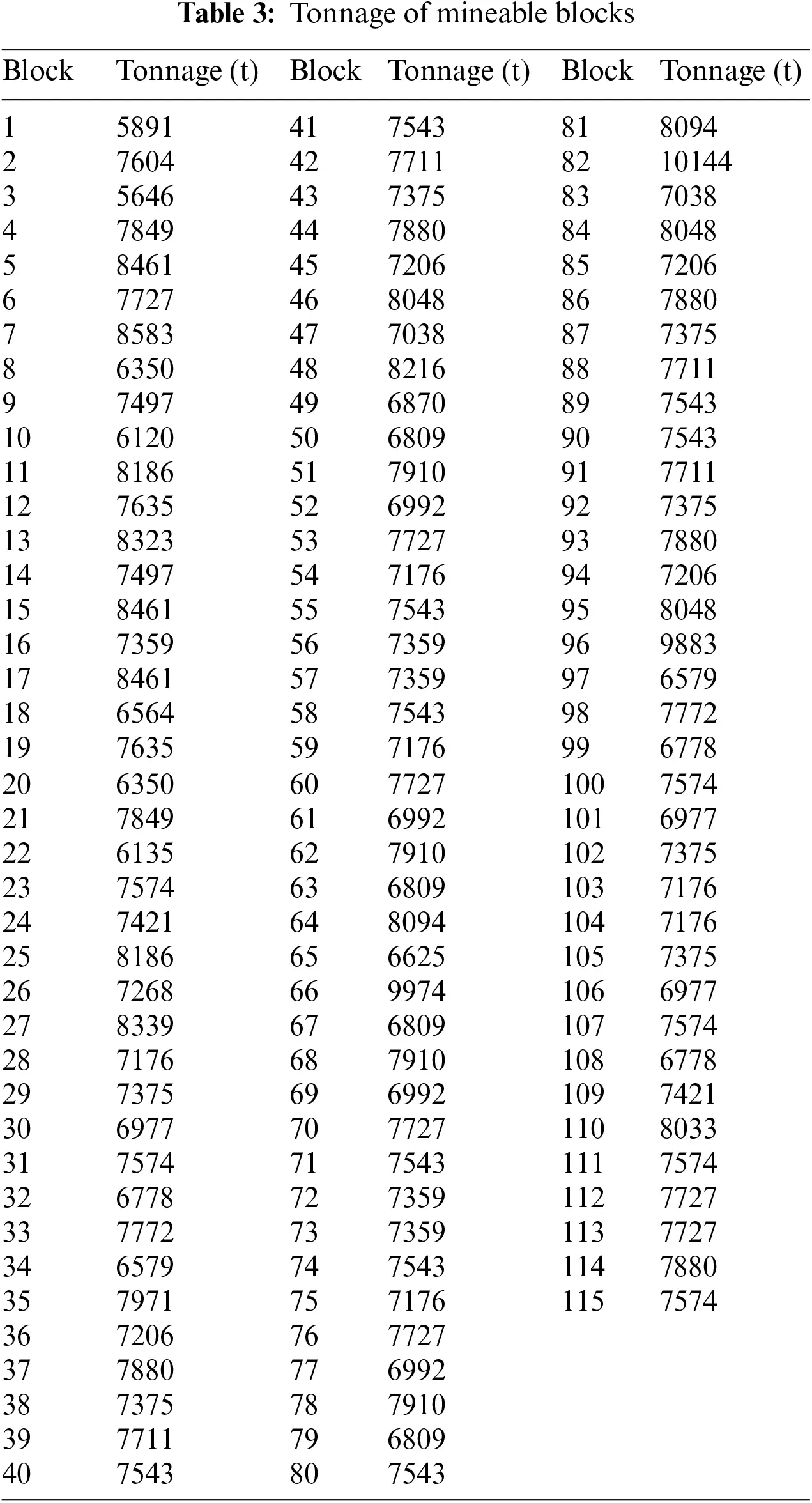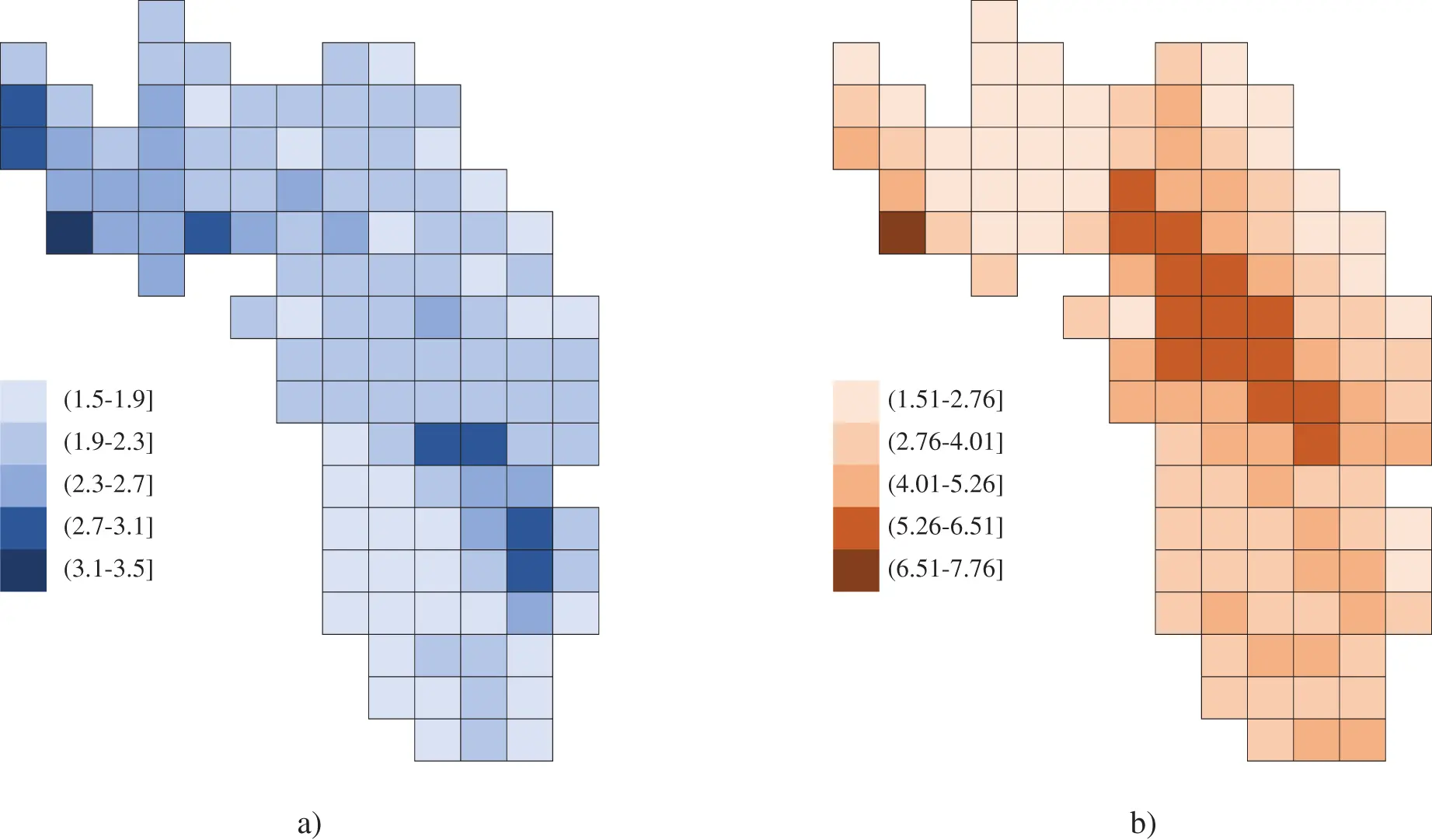The partitioning algorithm produced five TMCs whose characteristics are presented in Table 4, and Fig. 3.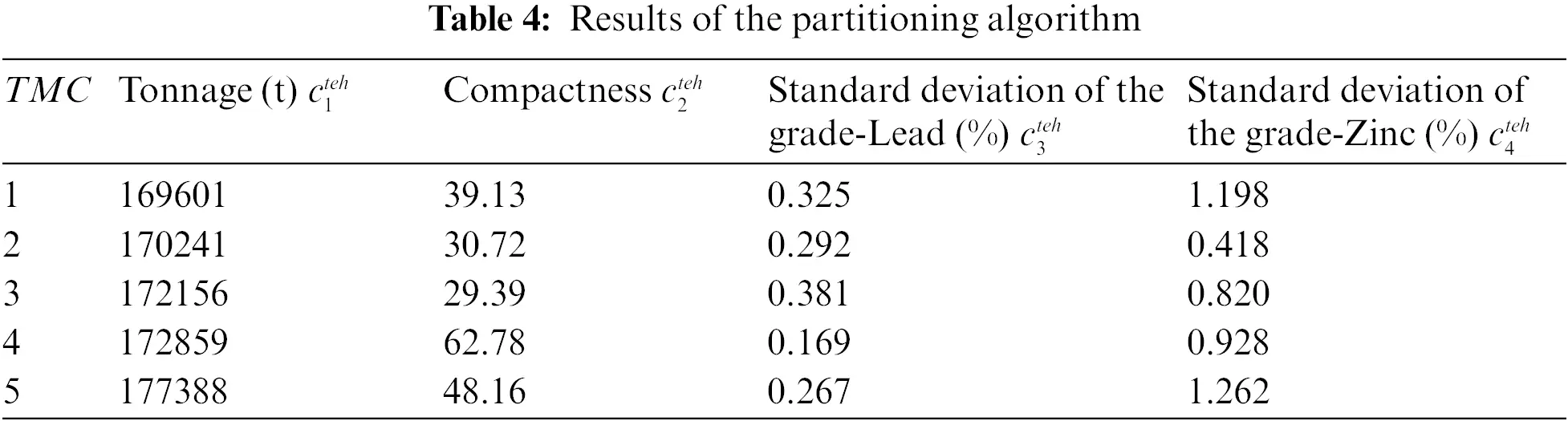Figure 3: TMCs obtained by the partitioning algorithm 

Since the results of the partitioning algorithm obtained in Table 4 meet the technological requirements, they actually represent the values of required technological vectors. Accordingly, the ADM matrix is created in the following form:

The efficiency evaluation of the algorithm is performed through the following steps:

Step 1: The detailed calculation process of the weights of criteria by the SPC method over the ADM matrix is demonstrated Step by Step.

Step 1.1: The elements of an artificial decision matrix are represented as follows:

DM=|xij|5×4=|A/CC1C2C3C4A116960139.130.3251.198A217024130.720.2920.418A317215629.390.3810.820A417285962.780.1690.928A517738848.160.2671.262|(26)

Step 1.2: The calculation of the symmetry point of each criterion is represented in Table 5.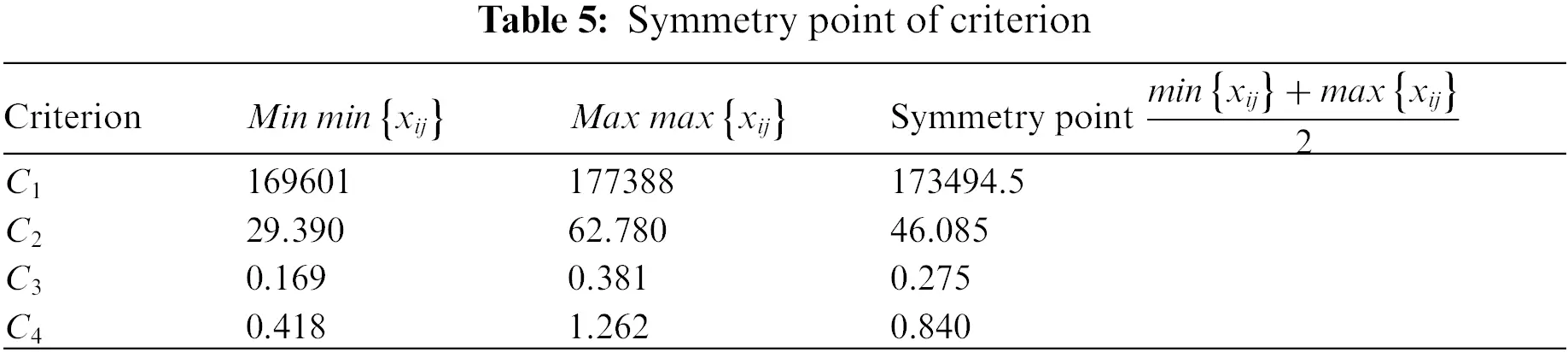Step 1.3: The resulting matrix of absolute distances is as follows:

D=|A/CC1C2C3C4A1|169601173494.5||39.1346.085||0.3250.275||1.1980.840|A2|170241173494.5||30.7246.085||0.2920.275||0.4180.840|A3|172156173494.5||29.3946.085||0.3810.275||0.8200.840|A4|172859173494.5||62.7846.085||0.1690.275||0.9280.840|A5|177388173494.5||48.1646.085||0.2670.275||1.2620.840||(27)

D=|A/CC1C2C3C4A13893.56.9550.0500.358A23253.515.3650.0170.422A31338.516.6950.1060.020A4635.516.6950.1060.088A53893.52.0750.0080.422|(28)

Step 1.4: Elements x11 and x12 of the matrix of the moduli of symmetry are calculated in the following way:

x11=3893.5+3253.5+1338.5+635.5+3893.55169601=0.0153;x12=6.955+15.365+16.695+16.695+2.075539.13=0.2953(29)

Analogically, we obtain values for the remaining elements, and the matrix of the moduli of symmetry is as follows:

R=|A/CC1C2C3C4A10.01530.29530.17660.2187A20.01530.37620.19660.6268A30.01510.39320.15070.3195A40.01510.18410.33960.2823A50.01470.24000.21500.2076|(30)

Step 1.5: The modulus of the symmetry of the first criterion C1 is calculated as follows:

q11=0.0153+0.0153+0.0151+0.0151+0.01475=0.0151(31)

The remaining moduli of symmetry are calculated in a similar way, and values are represented by the following vector Q:

Q=|q15|1×5=|0.01510.29780.21570.3310|(32)

Step 1.6: Weight of the first criterion C1 is:

w1=0.01510.0151+0.2978+0.2157+0.3310=0.0176(33)

The weights of criteria for the artificial MCDM problem are shown in Table 6, and Fig. 4.Figure 4: Weights of criteria calculated by the SPC method

Step 2: The values of criteria weights calculated by the SPC method are presented in Table 13. The weights of the optimal criteria are shown in Table 7, and Fig. 5.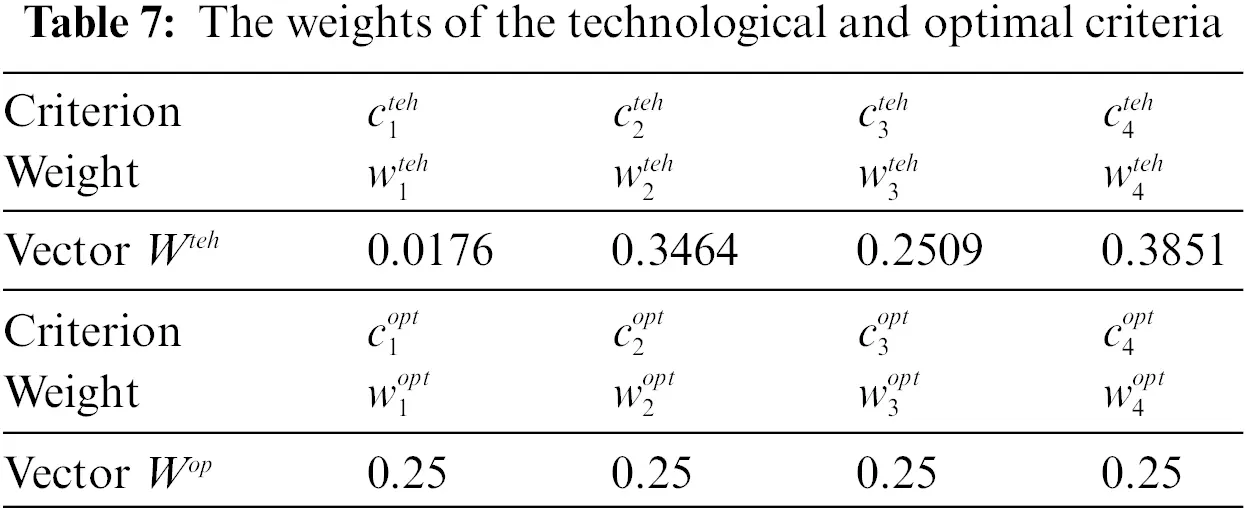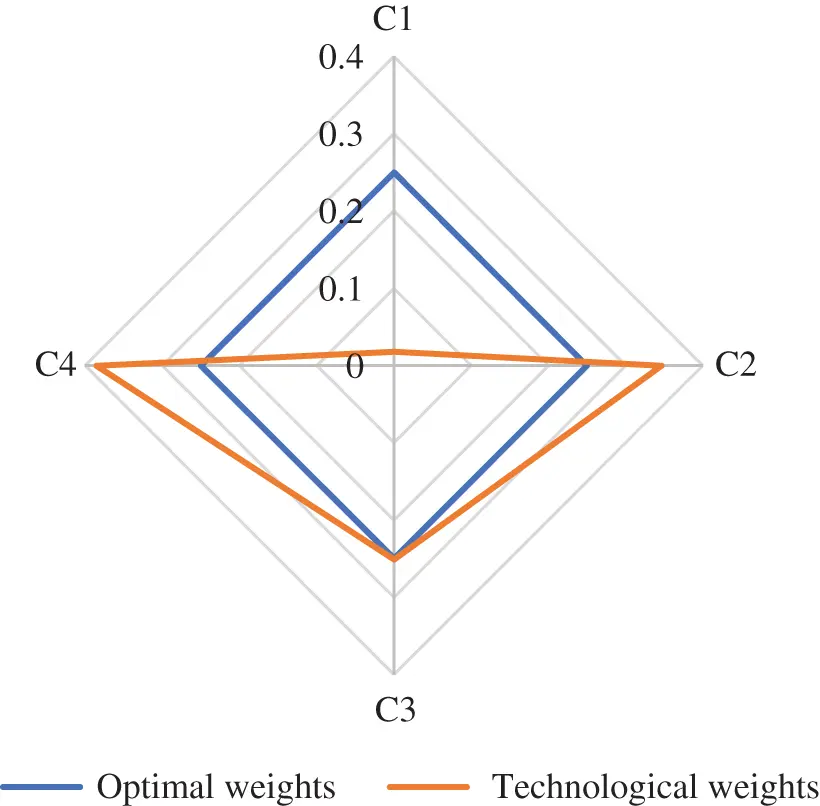Figure 5: Optimal and technological weights of criteria

Step 3: The entropy of the calculated weights and optimal weights is computed as follows:

Hteh=j=1Kwjtehlog(wjteh)=(0.0176log(0.0176))+(0.3464log(0.3464))+(0.2509log(0.2509))+(0.3851log(0.3851))=0.5006(34)

Hopt=j=1Kwjoptlog(wjopt)=(0.25log(0.25))+(0.25log(0.25))+(0.25log(0.25))+(0.25log(0.25))=0.6021(35)

Step 4: The efficiency of the algorithm (EOA) is calculated as:

EOA=HtehHop×100%=0.50060.6021×100%=83.15%(36)

The mineral deposit used in the example can be treated as a very difficult environment for the process of partitioning, with respect to the deposit’s characteristics. The space distribution of ore grades, ore tonnage, and the shape of the deposit are extremely irregular. Bearing in mind the very rigorous technological requirements, it can be said that the mineral deposit partitioning problem is a complex task. Despite this, the algorithm succeeded in dividing the mineral deposit with EOA of 83.15%, i.e., the efficiency of the algorithm is at a very high level.

4  A Comparison of the Objective Weighting Methods for Determining the Weights of Criteria

This section is related to the comparative analysis to show the capability of the SPC method to define veritable weights of criteria. The proposed SPC method is compared with the most frequently applied approaches for determining criteria weights, such as the CRITIC [7,8,10], Entropy [7,8,50], Standard Deviation [7,10,50] and MEREC [13,14,51] methods. The CRITIC, Entropy, Standard Deviation and MEREC methods used linear normalized decision matrix data to compute the weights of the criteria.

Beside the SD method, the CRITIC and Entropy methods are conventional and widely implemented objective weighting methods that are commonly used for determining criteria weights. These well-known methods are quite simple and easy to understand for decision-makers. Although they require a relatively high level of mathematical calculation, their application is growing constantly. Consequently, weight coefficients obtained by these methods are very reliable and stable. An enormous number of authors have also applied these two methods as a benchmark to compare their new developed methods. Due to these significant specifics, we selected the CRITIC and Entropy methods for comparison with our novel SPC method. In contrast to these usual methods, we have utilized a relatively new objective weighting method for comparison known as the MEREC method. In that way, we obtained an effective verification and validation of our new proposed method.

Our improvement of the CRITIC method involves changing the normalization procedure of the input data. The CRITIC method is quite sensitive to the application of different normalization techniques. The high impact of the normalization of input data is reflected in the final rank of the weight coefficients. Instead of the standard linear max-min normalization technique used in the CRITIC method, we applied linear sum procedure and obtained much better results for criteria weights. Because of these limitations, several authors have developed new modified and extended versions of the classical CRITIC method to improve the final values of weight coefficients [1,2,52]. As can be seen from Fig. 6 and Tables 9 and 10, the CRITIC method has the lowest degree of correlation with all the applied objective weighting methods.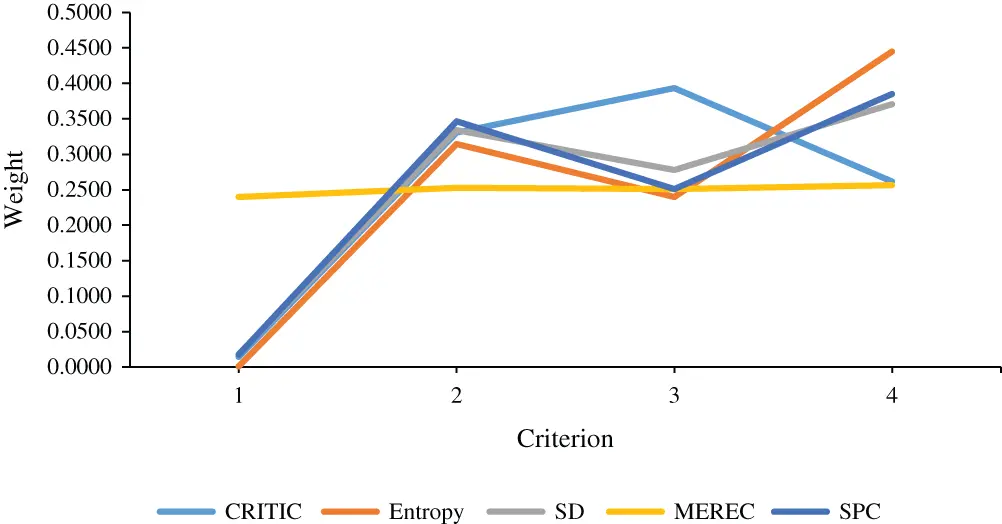Figure 6: Weights of criteria calculated by methods of comparison

Weights of criteria calculated by these methods are shown in Table 8 and in Fig. 6.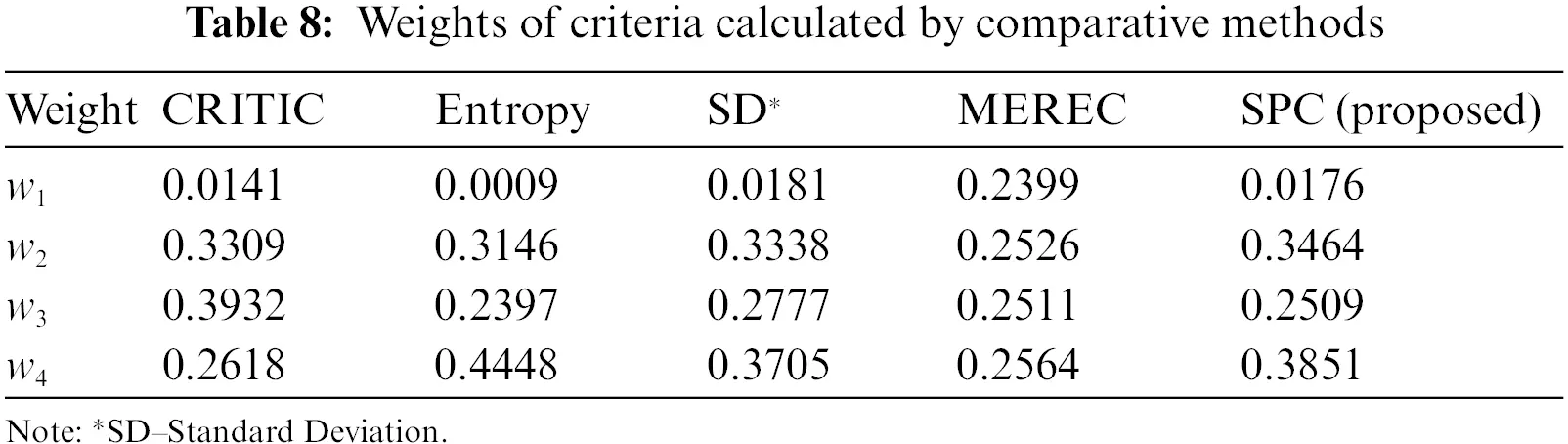The Pearson coefficient was used to define the degree of correlation between the weights of criteria computed by the CRITIC, Entropy, Standard Deviation, MEREC and SPC methods. The results of the comparative analysis are presented in Table 9.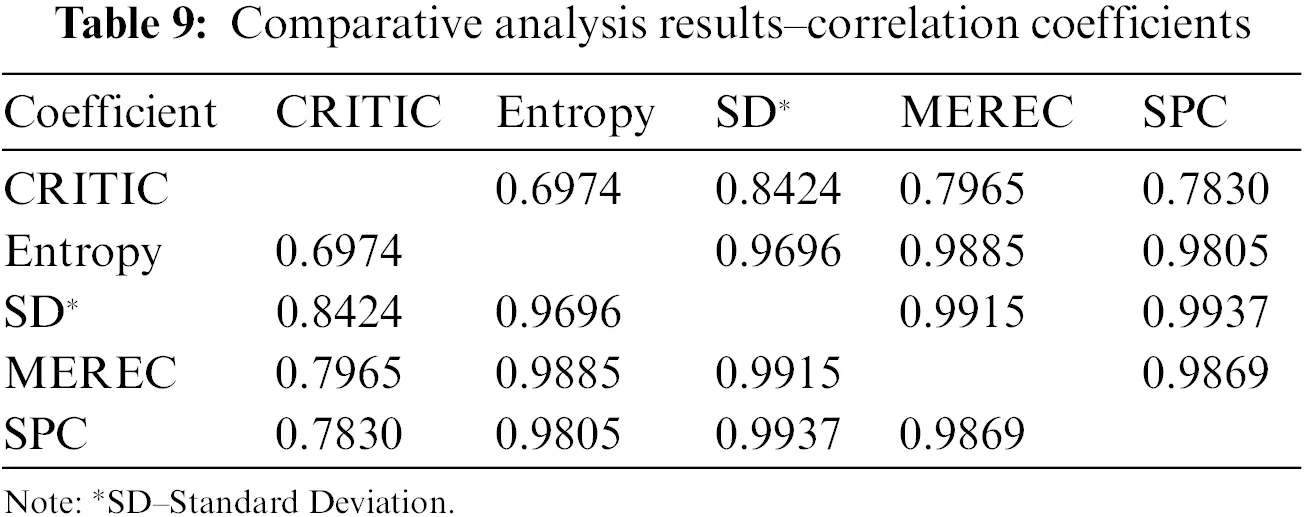Average correlation coefficients for each method of criteria weighting are shown in Table 10.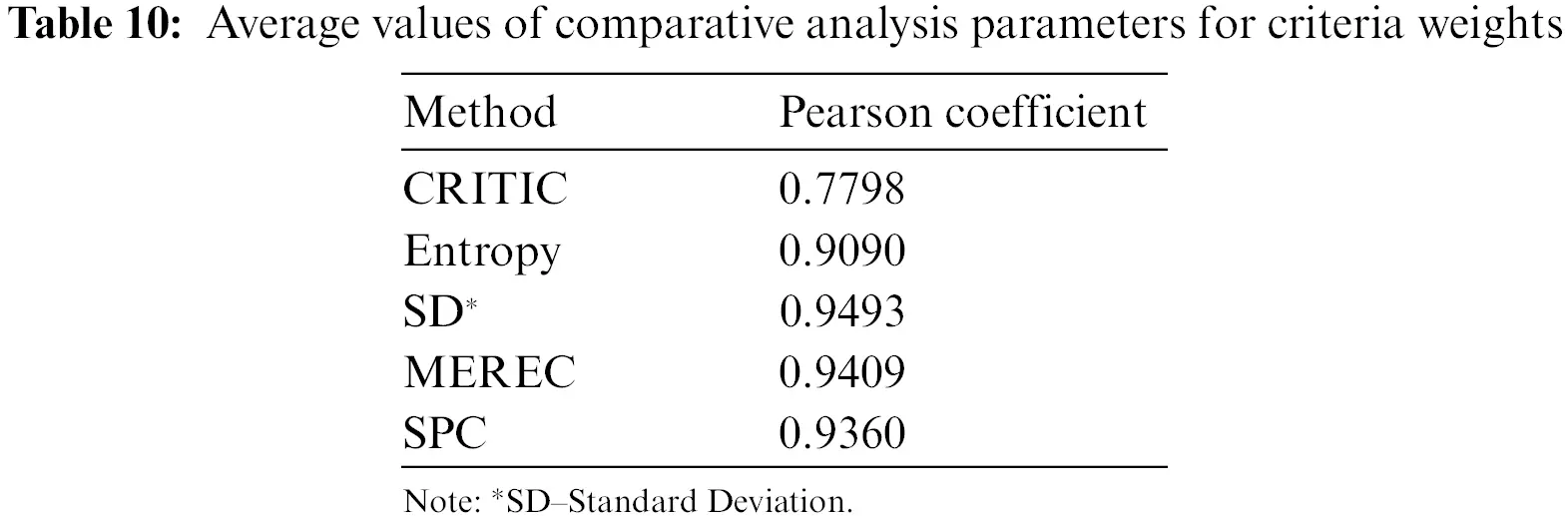Rank ordering of the weights of criteria for different criteria weighting methods is presented in Table 11 and Fig. 7.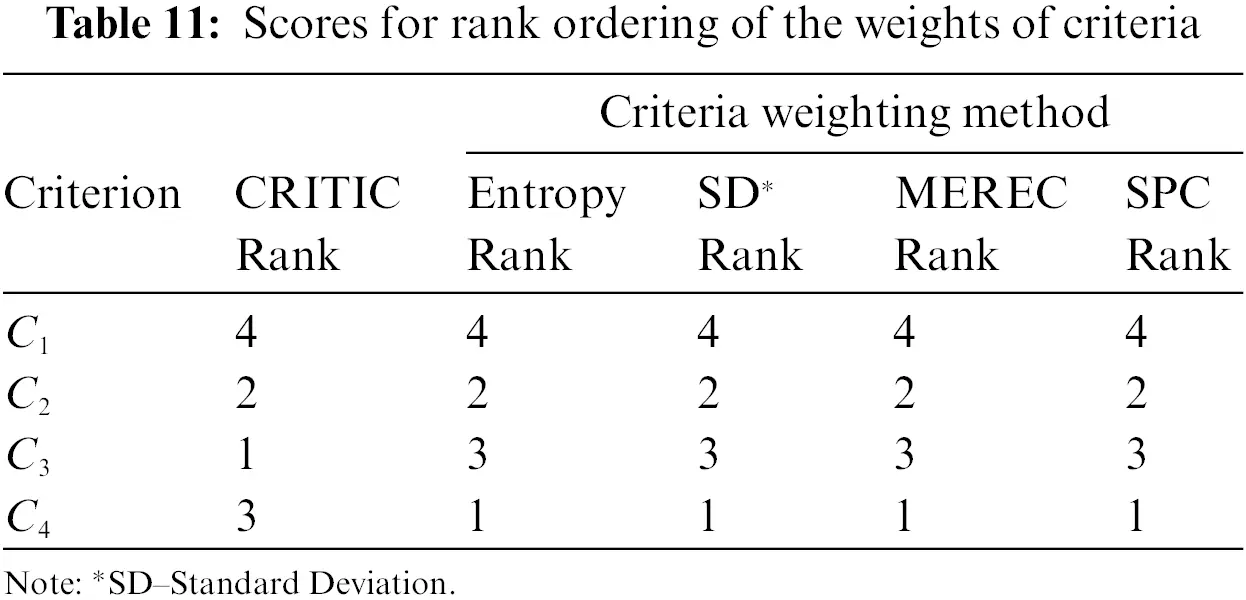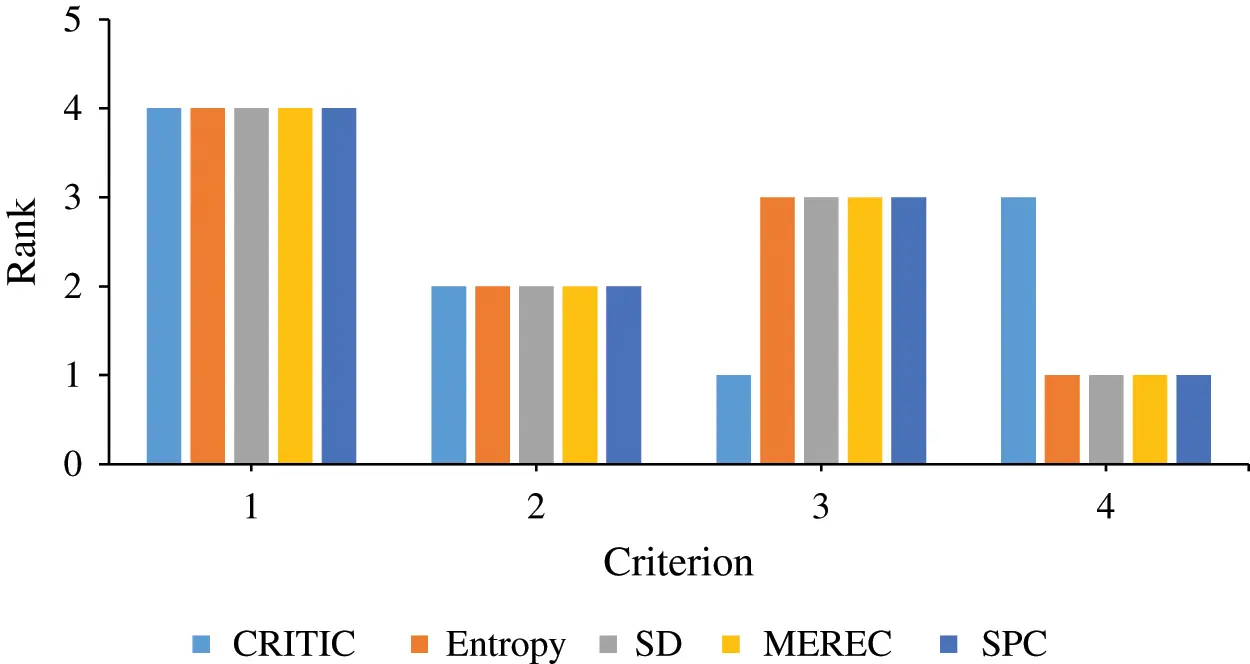Figure 7: Rank order of the weights of criteria

The same approach is used to make a comparative analysis for the rank ordering of the weights of criteria. The comparative analysis results are presented in Table 12.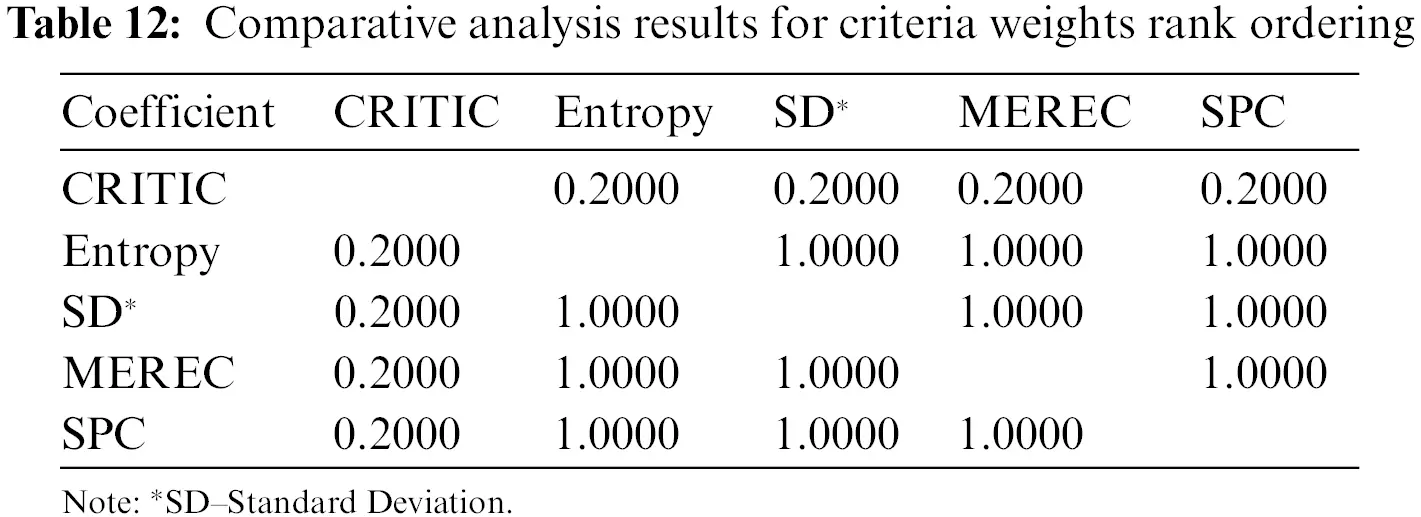The average correlation coefficients for each method of criteria rank ordering are shown in Table 13.The values shown in Table 9 indicate the existence of a strong correlation between the weights of criteria assessed by the SPC method and the weights assessed by the Entropy, Standard Deviation and MEREC methods. There is a slightly lower correlation between the SPC, Entropy, SD and MEREC methods on one side compared with the CRITIC method on the other. The average Pearson coefficient of correlation (0.9360; see Table 10) shows that the SPC method stands shoulder to shoulder with the other considered methods.

A comparative analysis using the criteria rank ordering is a more rigorous approach than the previous analysis. In such comparison circumstances, the SPC method showed very acceptable results. The correlation coefficient with the Entropy, Standard Deviation and MEREC methods is extremely high (1.000; see Table 12), while with the CRITIC method it is low (0.2000; see Table 12). The average coefficient of correlation of 0.8000 indicates the high level of applicability of the SPC method.

According to the above discussions, we can say that the SPC method is very capable of assessing the weights of criteria in MCDM problems. Furthermore, all the parameters of comparison showed that the developed method is very reliable.

The efficiency of the algorithm (EOA) by other objective weighting methods that are used for comparison analysis is calculated using the Eq. (24) and the results obtained are shown in Table 14 and Fig. 8.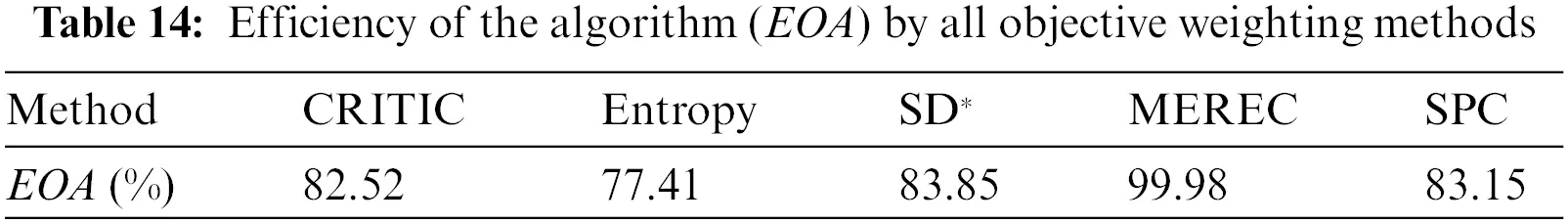The efficiency of the algorithm determined by all the applied objective weighting methods is shown in Table 14 and Fig. 8. From the obtained results, it is clearly visible that all methods have either a high and or high EOA value, according to Table 1. The MEREC method with 99.98% EOA indicates superiority over the other methods, although the weights of criteria are not graphically matched to other methods as can be seen from Fig. 6. However, the rank ordering of the weights of criteria obtained by the MEREC method has an extremely high correlation with other methods. The SPC method demonstrated the very high value of 83.15% EOA relative to the other methods (CRITIC 82.52%, Entropy 77.41% and SD 83.85% of EOA). Obviously, SPC is completely competitive with all methods and absolutely applicable in solving such a complex problem providing verification and validation of the partitioning algorithm.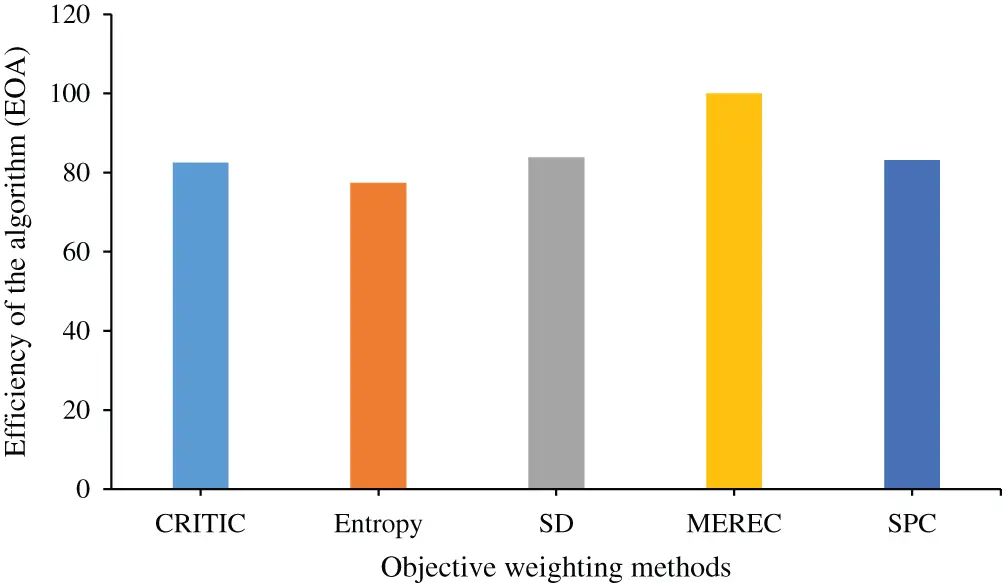Figure 8: Graphical review of the efficiency of the algorithm

From the extensive comparison analysis, we can highlight two main advantages of the new proposed SPC method. The first advantage is reflected in the fact that this method illustrates a new objective weighting method for defining the weights of criteria. Each MCDM technique should increase objectivity during the decision-making process. Since the attribute importance plays a very dominant role in the decision-making process, this proposed method helps decision-makers to obtain a more objective and reliable final rank of alternatives. The second advantage of the SPC method is expressed through the efficiency evaluation of the mineral deposit partitioning algorithm. This approach presents the novel mechanism for estimating the performance of every partitioning algorithm. It means that a uniform distribution of weighted coefficients indicates the high efficiency of the algorithm. As a very flexible and understandable approach, the SPC method can be extensively incorporated with conventional MCDM techniques for solving different problems. Certainly, the new proposed SPC method can be successfully implemented in numerous spheres of science. In a domain of technical sciences, this method can be applied in electrotechnical, mechanical, civil, traffic, energy and many other sectors of engineering. Beside this wide application in engineering fields, the SPC method can be efficiently used in various areas, such as economic fields, medical disciplines, social problems and even in political sciences.

5  Conclusions, Limitations and Future Research

Every real-life problem contains an important element faced through a decision-making challenge. Sometimes decision-makers must react very quickly in a short period of time and under uncertain conditions. Mining engineers are constantly faced with a huge number of challenges. Every investor and mining company management tends to create an optimal production plan as quickly as possible to begin mining activities. This optimal production plan is recognized as a crucial activity if mining companies are to make a profit in a very risky and volatile environment. The new proposed SPC method can be very useful for mining engineers in forming an optimal production plan. Although this method represents the verification of a previously developed partitioning algorithm, it can be applied as a tool for partitioning the mineral deposits in future studies.

The weights of criteria have a significant influence on the solving of multi-criteria problems. Besides the alternatives ranking method, the objective evaluation of criteria weights is also recognized as a very important activity. Excluding the subjectivity related to the preference of criteria should help in selecting the best alternative, i.e., in making the best decision. For that purpose, we have developed an objective method called Symmetry Point of Criteria (the SPC method). Basically, this method measures the absolute distance between the criterion value of a range of alternatives and the symmetry point of the criterion. The symmetry point represents the midpoint between two extreme criterion values (min and max value of the criterion). Every objective method can result in different values of criteria for individual decision-making problems but obtained values should be consistent as much as possible. Comparative analysis between the novel SPC method and the CRITIC, Entropy, Standard Deviation and MEREC methods shows that our method is capable of determining the criteria weights in a very efficient way. The average Pearson correlation coefficient of criteria rank ordering is 0.8000. The real-life applicability of the SPC method is demonstrated in the case of the mineral deposit multi-criteria partitioning algorithm. The efficiency of the algorithm depends directly on the values of the weights of criteria. If the calculated weights are mainly uniformly distributed, then the efficiency of the algorithm is higher.

The limitations of the proposed method are as follows. First of all, a hypothetical example is used to test the efficiency of the mineral deposit partitioning algorithm by the SPC method. Future research should be focused on implementing a real-life case study for testing the performance of the above-mentioned partitioning algorithm. Beside this obvious shortcoming, the non-inclusion of uncertainty theories into the initial decision-making matrix with input data is recognized as another limitation of the proposed method. Future research must include many different types of fuzzy, neutrosophic and intuitionistic numbers, creating an uncertain environment, and the final ranking of alternatives will be obtained under more reliable conditions. It is possible to interpret the limitations of the case study as follows. Firstly, only the basic attributes (four technological criteria) that characterize a mineral deposit are considered for the partitioning algorithm of the mineral deposit in this case study. In future works, there is a possibility to increase the number of criteria and test the efficiency of the partitioning algorithm by this proposed SPC method. Secondly, one of the key attributes that must meet the technological requirements is ore grade. Since the standard deviation is used to calculate ore grade, involving fuzzy numbers in the process of calculating the standard deviation of the ore grade is a very difficult and complex task. Future work can be directed towards overcoming this problem in the calculation process of standard deviation under a fuzzy environment by developing software for that purpose.

If we take into consideration that many real-life multi-criteria decision-making problems are burdened by uncertainties, then future research will be directed to including them in the SPC method. We will also explore how the developed method behaves in such environments. An extended version of our paper can be performed by introducing the stochastic diffusion process for the purpose of describing the features of some criteria. In that way, we can develop a dynamic model that is capable of solving more complex problems in the real world. Another variant of upgrading the paper should be related to integrating the SPC method with other MCDM processes. Certainly, there is potential to combine our developed SPC method with other subjective and objective weighting methods producing a new hybrid approach for calculating the weights of criteria.

Funding Statement: The authors received no specific funding for this study.

Conflicts of Interest: The authors declare that they have no conflicts of interest to report regarding the present study.

## References

1. Krishnan, A. R., Kasim, M. M., Hamid, R., & Ghazali, M. F. (2021). A modified CRITIC method to estimate the objective weights of decision criteria. Symmetry, 13(6), 973. [Google Scholar] [CrossRef]
2. Žižović, M., Miljković, B., & Marinković, D. (2020). Objective methods for determining criteria weight coefficients: A modification of the CRITIC method. Decision Making: Applications in Management and Engineering, 3(2), 149-161. [Google Scholar]
3. Cavallaro, F., Zavadskas, E. K., & Raslanas, S. (2016). Evaluation of combined heat and power (CHP) systems using fuzzy shannon entropy and fuzzy TOPSIS. Sustainability, 8(6), 556. [Google Scholar] [CrossRef]
4. Wang, R., Li, X., & Li, C. (2021). Optimal selection of sustainable battery supplier for battery swapping station based on triangular fuzzy entropy-MULTIMOORA method. Journal of Energy Storage, 34, 102013. [Google Scholar] [CrossRef]
5. Chodha, V., Dubey, R., Kumar, R., Singh, S., & Kaur, S. (2022). Selection of industrial arc welding robot with TOPSIS and entropy MCDM techniques. Materials Today: Proceedings, 50, 709-715. [Google Scholar] [CrossRef]
6. Vavrek, R., & Bečica, J. (2020). Efficiency evaluation of cultural services in the Czech Republic via multi-criteria decision analysis. Sustainability, 12(8), 3409. [Google Scholar] [CrossRef]
7. Mukhametzyanov, I. (2021). Specific character of objective methods for determining weights of criteria in MCDM problems: Entropy, CRITIC and SD. Decision Making: Applications in Management and Engineering, 4(2), 76-105. [Google Scholar] [CrossRef]
8. Vujičić, M. D., Papić, M. Z., & Blagojević, M. D. (2017). Comparative analysis of objective techniques for criteria weighing in two MCDM methods on example of an air conditioner selection. Tehnika, 72(3), 422-429. [Google Scholar] [CrossRef]
9. Yalcin, N., & Ünlü, U. (2018). A multi-criteria performance analysis of initial public offering (IPO) firms using CRITIC and VIKOR methods. Technological and Economic Development of Economy, 24, 534-560. [Google Scholar] [CrossRef]
10. Şahin, M. (2020). Hybrid multiattribute decision method for material selection. International Journal of Pure and Applied Sciences, 6(2), 107-117. [Google Scholar]
11. Wei, G., Lei, F., Lin, R., Wang, R., & Wei, Y. (2020). Algorithms for probabilistic uncertain linguistic multiple attribute group decision making based on the GRA and CRITIC method: Application to location planning of electric vehicle charging stations. Economic Research-Ekonomska Istraživanja, 33(1), 828-846. [Google Scholar] [CrossRef]
12. Mešić, A., Miškić, S., Stević, Ž., & Mastilo, Z. (2022). Hybrid MCDM solutions for evaluation of the logistics performance index of the western Balkan countries. Economics-Innovative and Economics Research Journal, 10(1), 13-34. [Google Scholar]
13. Keshavarz-Ghorabaee, M., Amiri, M., Zavadskas, E. K., Turskis, Z., & Antucheviciene, J. (2021). Determination of objective weights using a new method based on the removal effects of criteria (MEREC). Symmetry, 13(4), 525. [Google Scholar] [CrossRef]
14. Hadi, A., & Abdullah, M. Z. (2022). Web and IoT-based hospital location determination with criteria weight analysis. Bulletin of Electrical Engineering and Informatics, 11(1), 386-395. [Google Scholar] [CrossRef]
15. Alao, M. A., Popoola, O. M., & Ayodele, T. R. (2021). Selection of waste-to-energy technology for distributed generation using IDOCRIW-weighted TOPSIS method: A case study of the city of Johannesburg, South Africa. Renewable Energy, 178, 162-183. [Google Scholar] [CrossRef]
16. Podvezko, V., Zavadskas, E. K., & Podviezko, A. (2020). An extension of the new objective weight assessment methods CILOS and IDOCRIW to fuzzy MCDM. Economic Computation & Economic Cybernetics Studies & Research, 54(2), 59-75. [Google Scholar]
17. Podvezko, V., Kildienė, S., & Zavadskas, E. K. (2017). Assessing the performance of the construction sectors in the Baltic states and Poland. Panoeconomicus, 64(4), 493-512. [Google Scholar] [CrossRef]
18. Gorcun, O. F., Senthil, S., & Küçükönder, H. (2021). Evaluation of tanker vehicle selection using a novel hybrid fuzzy MCDM technique. Decision Making: Applications in Management and Engineering, 4(2), 140-162. [Google Scholar] [CrossRef]
19. Cakar, T., & Çavuş, B. (2021). Supplier selection process in dairy industry using fuzzy TOPSIS method. Operational Research in Engineering Sciences: Theory and Applications, 4(1), 82-98. [Google Scholar] [CrossRef]
20. Pamučar, D., Stević, Ž., & Sremac, S. (2018). A new model for determining weight coefficients of criteria in MCDM models: Full consistency method (FUCOM). Symmetry, 10(9), 393. [Google Scholar] [CrossRef]
21. Stanujkic, D., Zavadskas, E. K., Karabasevic, D., Smarandache, F., & Turskis, Z. (2017). The use of the pivot pairwise relative criteria importance assessment method for determining the weights of criteria. Infinite Study, Romanian Journal of Economic Forecasting, 20(4), 116-133. [Google Scholar]
22. Keršulienė, V., Zavadskas, E. K., & Turskis, Z. (2010). Selection of rational dispute resolution method by applying new step-wise weight assessment ratio analysis (SWARA). Journal of Business Economics and Management, 11(2), 243-258. [Google Scholar] [CrossRef]
23. Krylovas, A., Zavadskas, E. K., Kosareva, N., & Dadelo, S. (2014). New KEMIRA method for determining criteria priority and weights in solving MCDM problem. International Journal of Information Technology & Decision Making, 13(6), 1119-1133. [Google Scholar] [CrossRef]
24. Wind, Y., & Saaty, T. L. (1980). Marketing applications of the analytic hierarchy process. Management Science, 26(7), 641-658. [Google Scholar] [CrossRef]
25. Roszkowska, E. (2013). Rank ordering criteria weighting methods–A comparative overview. Optimum. Studia Ekonomiczne, 5(65), 14-33. [Google Scholar] [CrossRef]
26. Patel, M. R., Vashi, M. P., Bhatt, B. V. (2017). SMART-multi-criteria decision-making technique for use in planning activities. New Horizons in Civil Engineering (NHCE 2017), pp. 1–6. Surat, Gujarat, India.
27. Krylovas, A., Kosareva, N., & Zavadskas, E. K. (2018). WEBIRA-comparative analysis of weight balancing method. International Journal of Computers, Communications & Control, 12(2), 238-253. [Google Scholar]
28. Pamucar, D., Deveci, M., Gokasar, I., Tavana, M., & Köppen, M. (2022). A metaverse assessment model for sustainable transportation using ordinal priority approach and Aczel-Alsina norms. Technological Forecasting and Social Change, 182, 121778. [Google Scholar] [CrossRef]
29. Odu, G. O. (2019). Weighting methods for multi-criteria decision-making technique. Journal of Applied Sciences and Environmental Management, 23(8), 1449-1457. [Google Scholar] [CrossRef]
30. Jahan, A., Mustapha, F., Sapuan, S. M., Ismail, M. Y., & Bahraminasab, M. (2012). A framework for weighting of criteria in ranking stage of material selection process. The International Journal of Advanced Manufacturing Technology, 58(1), 411-420. [Google Scholar] [CrossRef]
31. Chen, C. H. (2020). A novel multi-criteria decision-making model for building material supplier selection based on entropy-AHP weighted TOPSIS. Entropy, 22(2), 259. [Google Scholar] [CrossRef]
32. Ali, T., Chiu, Y. R., Aghaloo, K., Nahian, A. J., & Ma, H. (2020). Prioritizing the existing power generation technologies in Bangladesh’s clean energy scheme using a hybrid multi-criteria decision-making model. Journal of Cleaner Production, 267, 121901. [Google Scholar] [CrossRef]
33. Zavadskas, E. K., Cavallaro, F., Podvezko, V., Ubarte, I., & Kaklauskas, A. (2017). MCDM assessment of a healthy and safe built environment according to sustainable development principles: A practical neighborhood approach in vilnius. Sustainability, 9(5), 702. [Google Scholar] [CrossRef]
34. Stanković, M., Stević, Ž., Das, D. K., Subotić, M., & Pamučar, D. (2020). A new fuzzy MARCOS method for road traffic risk analysis. Mathematics, 8(3), 457. [Google Scholar] [CrossRef]
35. Boral, S., Howard, I., Chaturvedi, S. K., McKee, K., & Naikan, V. N. A. (2020). An integrated approach for fuzzy failure modes and effects analysis using fuzzy AHP and fuzzy MAIRCA. Engineering Failure Analysis, 108, 104195. [Google Scholar] [CrossRef]
36. Žižović, M., Pamučar, D., Albijanić, M., Chatterjee, P., & Pribićević, I. (2020). Eliminating rank reversal problem using a new multi-attribute model—The RAFSI method. Mathematics, 8(6), 1015. [Google Scholar] [CrossRef]
37. Nunić, Z. (2018). Evaluation and selection of the PVC carpentry manufacturer using the FUCOM-MABAC model. Operational Research in Engineering Sciences: Theory and Applications, 1(1), 13-28. [Google Scholar]
38. Tyagi, M., Panchal, D., Kumar, D., & Walia, R. S. (2021). Modeling and analysis of lean manufacturing strategies using ISM-fuzzy MICMAC approach. Operational Research in Engineering Sciences: Theory and Applications, 4(1), 38-66. [Google Scholar] [CrossRef]
39. Wu, Q., Liu, X., Qin, J., Zhou, L., & Mardani, A. (2022). An integrated generalized TODIM model for portfolio selection based on financial performance of firms. Knowledge-Based Systems, 249, 108794. [Google Scholar] [CrossRef]
40. Sahu, R., Dash, S. R., & Das, S. (2021). Career selection of students using hybridized distance measure based on picture fuzzy set and rough set theory. Decision Making: Applications in Management and Engineering, 4(1), 104-126. [Google Scholar] [CrossRef]
41. Riaz, M., Garg, H., Farid, H. M. A., & Chinram, R. (2021). Multi-criteria decision making based on bipolar picture fuzzy operators and new distance measures. Computer Modeling in Engineering & Sciences, 127(2), 771-800. [Google Scholar] [CrossRef]
42. Rogulj, K., Kilić Pamuković, J., & Ivić, M. (2021). Hybrid MCDM based on VIKOR and cross entropy under rough neutrosophic set theory. Mathematics, 9(12), 1334. [Google Scholar] [CrossRef]
43. Du, C. G., & Ye, J. (2021). Weighted parameterized correlation coefficients of indeterminacy fuzzy multisets and their multicriteria group decision making method with different decision risks. Computer Modeling in Engineering & Sciences, 129(1), 341-354. [Google Scholar] [CrossRef]
44. Aikhuele, D. O. (2018). Intuitionistic fuzzy model for reliability management in wind turbine system. Applied Computing and Informatics, 16(1/2), 181-194. [Google Scholar] [CrossRef]
45. Xia, S., Yang, H., & Chen, L. (2021). An incomplete soft set and its application in MCDM problems with redundant and incomplete information. International Journal of Applied Mathematics and Computer Science, 31, 417-430. [Google Scholar]
46. Gligorić, M. V., Gligorić, Z. M., Beljić, Č. R., Lutovac, S. M., & Damnjanović, V. M. (2019). Long-term room and pillar mine production planning based on fuzzy 0–1 linear programming and multicriteria clustering algorithm with uncertainty. Mathematical Problems in Engineering, 2019, [Google Scholar] [CrossRef]
47. https://tselab.stanford.edu/mirror/ee376a_winter1617/Lecture_15.pdf.
48. Shannon, C. E. (1948). A mathematical theory of communication. The Bell System Technical Journal, 27(3), 379-423. [Google Scholar] [CrossRef]
49. Schwartzberg, J. E. (1966). Reapportionment, gerrymanders, and the notion of compactness. Minnesota Law Review, 50, 443-452. [Google Scholar]
50. Zardari, N. H., Ahmed, K., Shirazi, S. M., Yusop, Z. B. (2015). Weighting methods and their effects on multi-criteria decision-making model outcomes in water resources management. Berlin/Heidelberg, Germany: Springer.
51. Trung, D. D., & Thinh, H. X. (2021). A multi-criteria decision-making in turning process using the MAIRCA, EAMR, MARCOS and TOPSIS methods: A comparative study. Advances in Production Engineering & Management, 16(4), 443-456. [Google Scholar] [CrossRef]
52. Wu, D., Yan, J., Wang, M., Chen, G., & Jin, J. (2022). Multidimensional connection cloud model coupled with improved CRITIC method for evaluation of eutrophic water. Mathematical Problems in Engineering, 2022, [Google Scholar] [CrossRef]

Gligorić, Z., Gligorić, M., Miljanović, I., Lutovac, S., Milutinović, A. (2023). Assessing Criteria Weights by the Symmetry Point of Criterion (Novel SPC Method)–Application in the Efficiency Evaluation of the Mineral Deposit Multi-Criteria Partitioning Algorithm. CMES-Computer Modeling in Engineering & Sciences, 136(1), 955–979.This work is licensed under a Creative Commons Attribution 4.0 International License , which permits unrestricted use, distribution, and reproduction in any medium, provided the original work is properly cited.

View

Like

## Related articles

• Hui Wang, Shuqi Wan
• Amar Prakash, Srinivasan, S. M.,...
• Z. Y. Yan, J. Zhang, W. Ye, T.X....
• J. Bielak, O. Ghattas, E.-J. Kim
• Hanbo Zhu, Changqing Miao, Meiling...